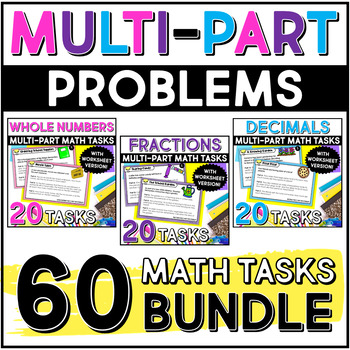# Multi-Part Math Constructed Response {Bundle}Subject
Resource Type
File Type
Zip (4 MB|180 (3 Levels Per Task))
Standards
\$6.00
Bundle
List Price:
\$12.00
You Save:
\$6.00
Products in this Bundle (3)
1. This resource contains 20 multi-part math constructed response tasks. 10 of the tasks are two part problems and 10 are three part problems. This resource includes whole number operations only (all four operations with a focus on multiplication and division).The tasks are differentiated with three di
2. This resource contains 20 multi-part math constructed response tasks. 10 of the tasks are two part problems and 10 are three part problems. This resource includes decimal operations only (all four operations). The tasks are differentiated with three different levels. The tasks themselves are not di
3. This resource contains 20 multi-part math constructed response tasks. 10 of the tasks are two part problems and 10 are three part problems. This resource includes fraction operations only (all four operations). The tasks are differentiated with three different levels. The tasks themselves are not d
• Bundle Description
• Standards

Need Multi Part math tasks to prepare your students for more rigorous state assessments?

This bundle includes 60 multi-part tasks: 20 with Fractions, 20 with Decimals, and 20 with Whole Numbers.

Click on the links to check out each resource in this bundle:

Multi-Part Constructed Response Resource for Fractions

Multi-Part Constructed Response Resource for Decimals

Multi-Part Constructed Response Resource for Whole Numbers

There are 30 two part tasks (10 for each skill) and 30 three part tasks (10 for each skill).

The task responses are differentiated with three different levels. The tasks themselves are not differentiated, however the student is given support to construct their answer. One version provides separate boxes for each part and sentence stems to help the student construct their answer. The second version provides separate boxes to help the students organize their thinking but no stems. The final version does not include separate boxes or stems.

*****************************************************************************

Thanks!

Jennifer Findley

Apply and extend previous understandings of division to divide unit fractions by whole numbers and whole numbers by unit fractions.
Solve real world problems involving multiplication of fractions and mixed numbers, e.g., by using visual fraction models or equations to represent the problem.
Apply and extend previous understandings of multiplication to multiply a fraction or whole number by a fraction.
Solve word problems involving addition and subtraction of fractions referring to the same whole, including cases of unlike denominators, e.g., by using visual fraction models or equations to represent the problem. Use benchmark fractions and number sense of fractions to estimate mentally and assess the reasonableness of answers. For example, recognize an incorrect result 2/5 + 1/2 = 3/7, by observing that 3/7 < 1/2.
Add and subtract fractions with unlike denominators (including mixed numbers) by replacing given fractions with equivalent fractions in such a way as to produce an equivalent sum or difference of fractions with like denominators. For example, 2/3 + 5/4 = 8/12 + 15/12 = 23/12. (In general, 𝘢/𝘣 + 𝘤/𝘥 = (𝘢𝘥 + 𝘣𝘤)/𝘣𝘥.)
Total Pages